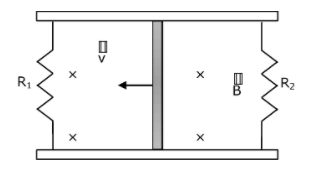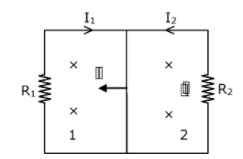A conducting bar of length L is free to slide on two parallel conducting rails as shown in the figureTwo resistors R1 and R2 are connected across the ends of the rails. There is a uniform magnetic field $$\vec{B}$$ pointing into the page. An external agent pulls the bar to the left at a constant speed v. The correct statement about the directions of induced currents I1 and I2 flowing through R1 and R2 respectively is :

a. I1 is in the clockwise direction and I2 is in the anticlockwise direction

b. Both I1 and I2 are in a clockwise direction

c. I1 is in the anticlockwise direction and I2 is in a clockwise direction

d. Both I1 and I2 are in the anticlockwise directionWhen the bar slides, the area of loop 1 decreases and that of loop 2 increases. Magnetic flux decreases in 1 and increases in 2. Therefore induced emf and current resist this change. As a result, B should increase in 1 and decrease in 2. So I1 should be clockwise and I2 anticlockwise.

Hence option (a) is correct.